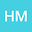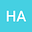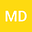Local discontinuous Galerkin method for distributed-order time-fractional diffusion-wave equation: Application of Laplace transform
•••• Mehdi Dehghan
Amirkabir University of Technology
Author ProfileAmirkabir University of Technology
Author ProfileMehdi Dehghan
Amirkabir University of Technology,
Author Profile## Abstract

In this paper, the Laplace transform combined with the local discontinuous Galerkin method is used for distributed-order time-fractional diffusion-wave equation. In this method,at first, we convert the equation to some time-independent problems by Laplace transform.Then we can solve these stationary equations by the local discontinuous Galerkin method to discretize diffusion operators at the same time. Then, by using a numerical inversion of the Laplace transform we can find the solutions of the original equation. One of the advantages of this procedure is its parallel implementation. Another advantage of this approach is that the number of stationary problems that should be solved is much less than that are needed in time-marching methods. Finally, some numerical experiments have been provided to show the accuracy and efficiency of the method.

#### Peer review status:ACCEPTED

24 Feb 2020Submitted to Mathematical Methods in the Applied Sciences
28 Feb 2020Submission Checks Completed
28 Feb 2020Assigned to Editor
09 Mar 2020Reviewer(s) Assigned
26 May 2020Review(s) Completed, Editorial Evaluation Pending
26 May 2020Editorial Decision: Revise Major